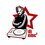# Area common to two regions

Consider the regions $(x,y)|\hspace{2mm}x^{2}+y^{2}\leq100$ and $sin(x+y)<0$.

Find the area of the region common to the above inequalities.Note by Krishna Jha
6 years, 10 months ago

This discussion board is a place to discuss our Daily Challenges and the math and science related to those challenges. Explanations are more than just a solution — they should explain the steps and thinking strategies that you used to obtain the solution. Comments should further the discussion of math and science.

When posting on Brilliant:

• Use the emojis to react to an explanation, whether you're congratulating a job well done , or just really confused .
• Ask specific questions about the challenge or the steps in somebody's explanation. Well-posed questions can add a lot to the discussion, but posting "I don't understand!" doesn't help anyone.
• Try to contribute something new to the discussion, whether it is an extension, generalization or other idea related to the challenge.

MarkdownAppears as
*italics* or _italics_ italics
**bold** or __bold__ bold
- bulleted- list
• bulleted
• list
1. numbered2. list
1. numbered
2. list
Note: you must add a full line of space before and after lists for them to show up correctly
paragraph 1paragraph 2

paragraph 1

paragraph 2

[example link](https://brilliant.org)example link
> This is a quote
This is a quote
    # I indented these lines
# 4 spaces, and now they show
# up as a code block.

print "hello world"
# I indented these lines
# 4 spaces, and now they show
# up as a code block.

print "hello world"
MathAppears as
Remember to wrap math in $$ ... $$ or $ ... $ to ensure proper formatting.
2 \times 3 $2 \times 3$
2^{34} $2^{34}$
a_{i-1} $a_{i-1}$
\frac{2}{3} $\frac{2}{3}$
\sqrt{2} $\sqrt{2}$
\sum_{i=1}^3 $\sum_{i=1}^3$
\sin \theta $\sin \theta$
\boxed{123} $\boxed{123}$

Sort by:

area of x^2+y^2 is 100π.sin(x+y)>0 which implies 0>x+y>2π.....like that split the parts in which sin(x+y)>0if you see the graph it form a symetry and if you fold the circle you can see common region is 50π.

- 6 years, 10 months ago

Yes, even I did think the same way...thanks for ur answer

- 6 years, 10 months ago

If $A_1 = \{(x,y)\,|\, x^2+y^2 \le 100 , \sin(x+y) > 0\}$ and $A_2 = \{(x,y) \,|\, x^2+y^2 \le 100: \sin(x+y) < 0\}$, then a rotation through $\pi$ about the origin maps $A_1$ onto $A_2$, and vice versa. Thus $A_1$ and $A_2$ have the same area. The sets are disjoint, and their union is the interior of the circle (with $9$ lines removed), and so the area of each is $\tfrac12 \times 10^2\pi = 50\pi$.

- 6 years, 10 months ago

Thanks!!!.

- 6 years, 10 months ago

Hello Mark!

What does this statement mean: "then a rotation through $π$ about the origin maps $A_1$ onto $A_2$, and vice versa."? Can you please post a link which explains this?

Thanks!

- 6 years, 10 months ago

The point $(x,y)$ belongs to $A_1$ if and only if the point $(-x,-y)$ belongs to $A_2$. Changing the sign of both $x$ and $y$ is achieved by a rotation about the origin through $\pi$ (or successive reflections in the $x$- and $y$-axes, or an enlargement scale factor $-1$ through the origin). However you describe this transformation of the plane $\mathbb{R}^2$, it preserves areas, so that $A_1$ and $A_2$ have the same area.

- 6 years, 10 months ago

Thanks Mark! :)

- 6 years, 10 months ago

Great work!!

- 6 years, 10 months ago

Nicely Done Mark !!

- 6 years, 10 months ago